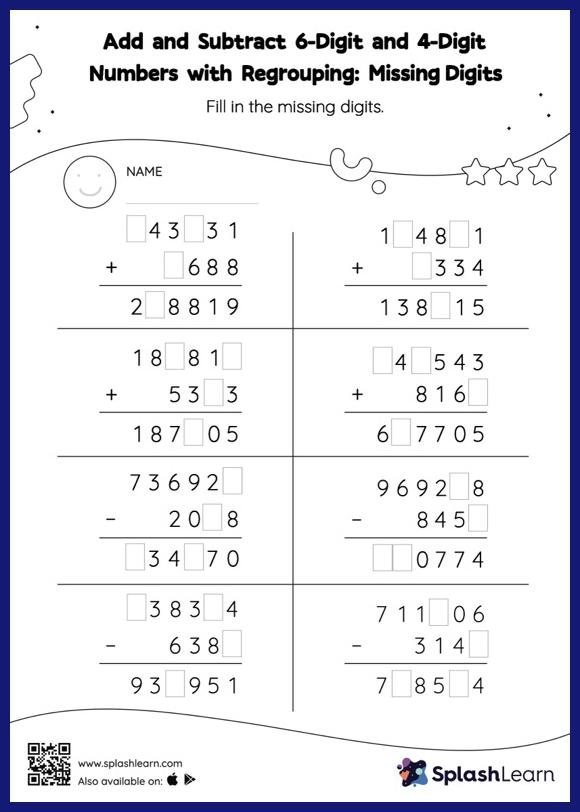# Add and Subtract 6-Digit and 4-Digit Numbers with Regrouping: Missing Digits Worksheet

Home > Add and Subtract 6-Digit and 4-Digit Numbers with Regrouping: Missing DigitsTo find the missing number on this worksheet, students can utilize the connection between addition and subtraction. In add and subtract 6-digit and 4-digit numbers with regrouping worksheet, they must additionally regroup numbers to reach the solution. In each problem, the numbers are laid out in the vertical format. In this format, pair of digits in each successive place are tackled one by one. This helps students follow a structured approach.